## Example Questions

### Example Question #1 : Simplifying Expressions

a # b = (a * b) + a

What is 3 # (4 # 1)?

20

15

12

27

8

27

Explanation:

Work from the "inside" outward.  Therefore, first solve 4 # 1 by replacing a with 4 and b with 1:

4 # 1 = (4 * 1) + 4 = 4 + 4 = 8

That means: 3 # (4 # 1) = 3 # 8.  Solve this now:

3 # 8 = (3 * 8) + 3 = 24 + 3 = 27

### Example Question #1 : Simplifying Expressions

Simplify the result of the following steps, to be completed in order:

2. Multiply the sum by 4

3. Add x to the product

4. Subtract x – y from the sum

28x + 11y

29x + 13y

28x + 12y

28x + 13y

28x – 13y

28x + 13y

Explanation:

Step 1: 7x + 3y

Step 2: 4 * (7x + 3y) = 28x + 12y

Step 3: 28x + 12y + x = 29x + 12y

Step 4: 29x + 12y – (x – y) = 29x + 12y – x + y = 28x + 13y

### Example Question #1 : Simplifying Expressions

Quantitative Comparison

x and y are non-zero integers.

Quantity A: (x – y)2

Quantity B: (x + y)2

Quantity B is greater.

Quantity A is greater.

The two quantities are equal.

The answer cannot be determined from the information given.

The answer cannot be determined from the information given.

Explanation:

Quantity A: (x – y)2 = x2 – 2xy + y2

Quantity B: (x + y)2 = x2 + 2xy + y2

Both have x2 + y2 so cancel those from both columns and just compare –2xy in Quantity A to 2xy in Quantity B. If x = 1 and y = 1, –2xy = –2 and 2xy = 2, so Quantity B is greater. But if x = 1 and y = –1, –2xy = 2 and 2xy = –2, so Quantity A is greater. The contradiction means the answer cannot be determined.

### Example Question #21 : Simplifying Expressions

Which is the greater quantity: the median of 5 positive sequential integers or the mean of 5 positive sequential integers?

The quantities are equal

The relationship cannot be determined

The mean is greater

The median is greater

The quantities are equal

Explanation:

If the first integer is, then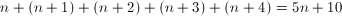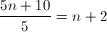This is the same as the median.

### Example Question #1 : How To Simplify An Expression

You are told thatcan be determined from the expression: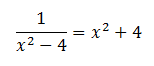Determine whether the absolute value ofis greater than or less than 2.

The relationship cannot be determined from the information given.

The quantities are equal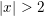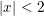Explanation:

The expression is simplified as follows: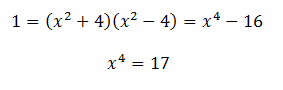Since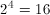the value ofmust be slightly greater for it to be 17 when raised to the 4th power.

### Example Question #1 : Simplifying Expressions

Quantitative Comparison

Quantity A: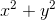Quantity B: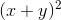Quantity B is greater.

The two quantities are equal.

Quantity A is greater.

The relationship cannot be determined from the information given.

The relationship cannot be determined from the information given.

Explanation:

(x + y)2 = x2 + 2xy + y2

Now, since there are no specifications on what x and y can equal, one or both of them could be 0, making the two columns equal. Any value other than 0 will make the columns unequal because of the additional 2xy term, so the answer cannot be determined.

### Example Question #1 : Simplifying Expressions

Which best describes the relationship between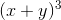and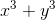if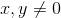?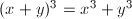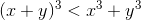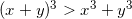The relationship cannot be determined from the information given.

The relationship cannot be determined from the information given.

Explanation:

Use substitution to determine the relationship.

For example, we could plug inand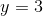.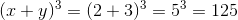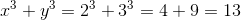So far it looks like the first expression is greater, but it's a good idea to try other values of x and y to be sure. This time, we'll try some negative values, say,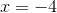and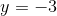.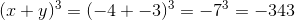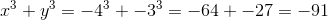This time the first quantity is smaller. Therefore the relationship cannot be determined from the information given.

### Example Question #1 : Simplifying Expressions

If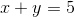and, then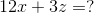Cannot be determinedExplanation:

We have three variables and only two equations, so we will not be able to solve for each independent variable. We need to think of another solution.

Notice what happens if we line up the two equations and add them together.

(x + y) + (3x – y + z) = 4x + z

and 5 + 3 = 8

Lets take this equation and multiply the whole thing by 3:

3(4x + z = 8)

Thus, 12x + 3z = 24.

### Example Question #2 : Simplifying Expressions

Quantitative Comparisonis an integer.

Quantity A: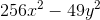Quantity B: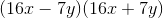Quantity A is greater.

Quantity B is greater.

The two quantities are equal.

The relationship cannot be determined from the information given.